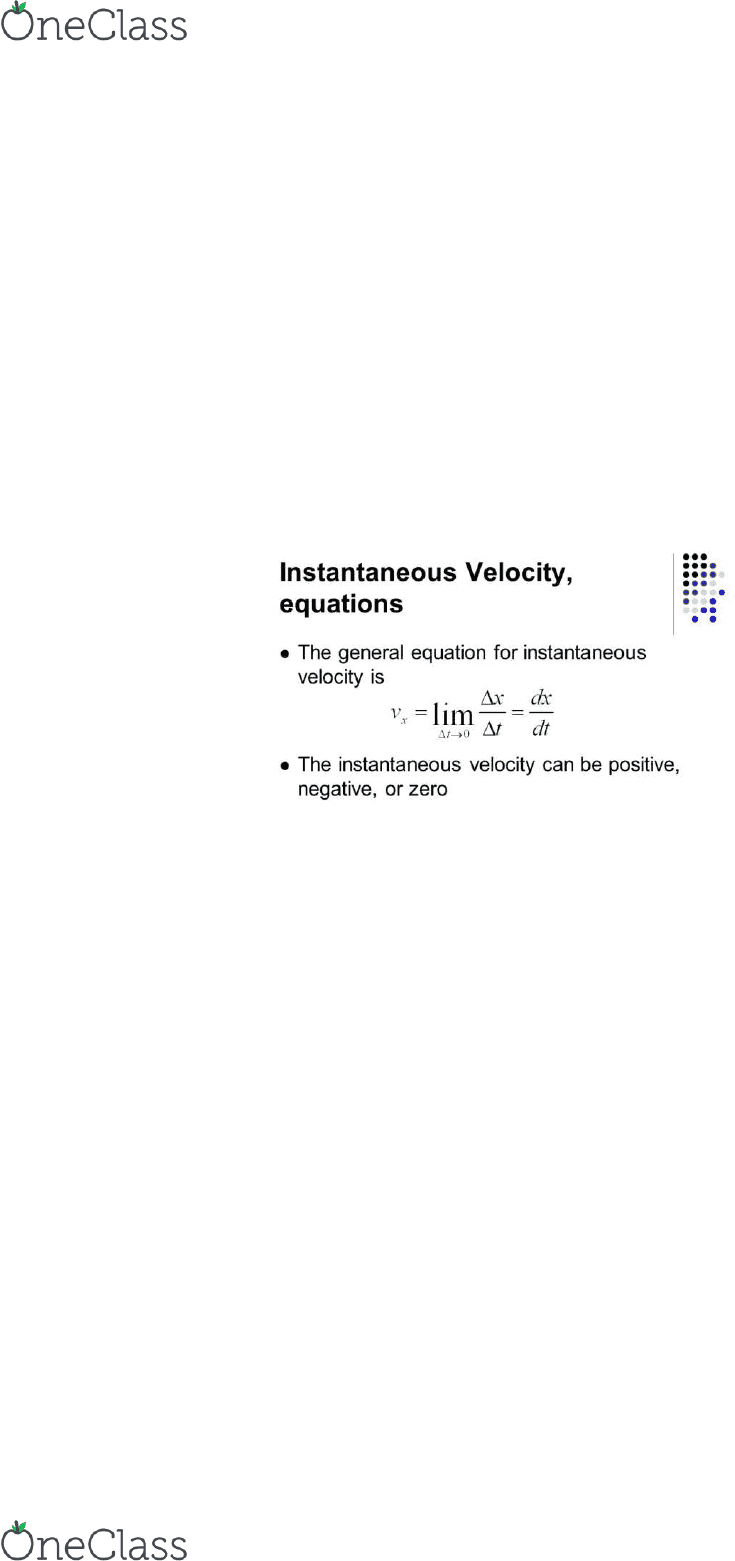# PHYS 1021 Lecture 1: Chapter 2

67 views3 pages2-1 Reference Frames and Displacement
1. Any kind of measurement regarding speed or distance but have a frame of reference
2. Direction of motion must be specified. Thus the use of the coordinate axes in physics
a. In one dimensional motion, only the x-axis is used to show position
b. The y-axis is used for when an object is dropped
3. Displacement
4. Vectors are used to show displacement: movement and magnitude
2-2 Average Velocity
1. Average speed: distance traveled/time
2. Velocity includes magnitude and direction divided by time
3. Average velocity: (final position initial position)/time
4. Elapsed time is always positive
2-3 Instantaneous Velocity
1. Instantaneous velocity: average velocity over an infinitely small time period
a.
b. Though t approaches 0, it should not be set as 0
c. Instantaneous speed is the same as instantaneous velocity
d. Average velocity is the same as instantaneous velocity if objects travel at
constant speed
e. On a graph of position vs time, slope is the change of Δx/Δt, it is also the
average velocity on the interval
f. The instantaneous velocity is equal to the slope of the tangent line of the curve
g. Acceleration is a vector as well
h. A object that is slowing down is decelerating
i. This is not the same as a negative acceleration which inidcates
direction
i. Instantaneous acceleration (a): the value of the average acceleration as Δt
approaches 0
find more resources at oneclass.com
find more resources at oneclass.com
Unlock document

This preview shows page 1 of the document.
Unlock all 3 pages and 3 million more documents.